## Guest

### Table of ContentRadioactivity was observed by Henri Becquerel in 1896 that some minerals like pitchblende emit radiation spontaneously, and this radiation can blacken photographic plates if photographic plate is wrapped in light proof paper. He called this phenomenon radioactivity. It was observed that radioactivity was not affected by temperature, pressure or chemical combination, it could therefore be a property that was intrinsic to the atom-more specifically to its nucleus. Detailed studies of radioactivity resulted in the discovery that radiation emerging from radioactive substances were of three types: α-rays, β-rays and γ-rays. Radioactivity is basically the process by which a nucleus of an unstable atom loses energy by emitting some particles. It is often termed as radioactive decay or nuclear decay. The material that spontaneously emits the three kinds of radiations alpha, beta and gamma rays is said to be a radioactive substance. Radioactive decay occurs in those nuclei which are unstable i.e. which don’t have sufficient amount of binding energy to hold the nucleus together due to the presence of excess number of protons or neutrons.

Whenever a substance undergoes radioactivity, there is a possibility of emission of three kinds of radiations- alpha, beta and gamma rays.These are the first three letters of the Greek alphabet. We discuss these in detail one by one.

## Alpha Rays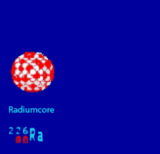Alpha rays are positively charged. An alpha particle, denoted by the symbol α, is a helium nucleus. A helium atom which has lost two electrons and carries a charge of +2e is called the alpha particle. Its mass is about four times that of a hydrogen atom. Initially, it escapes from the nucleus of its parent atom and is further repelled by electromagnetism, since both the alpha particles as well as the nucleus are positively charged. The velocity of α-particles ranges from 5 to 7 percent of the velocity of light. These have very little penetrating power into a material medium but have a very high ionizing power.

After the emission of an alpha particle, the original atom gets transformed into a new element. While its atomic number gets reduced by two, the mass number reduces by four. We consider the example of uranium-238 involving the emission of alpha particle: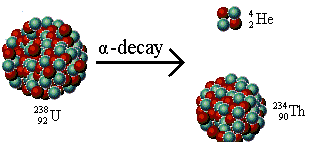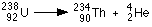Alpha particles have the least penetrating power. They do not travel very far in air and cannot even penetrate a piece of paper. Before they can use any kind of harm, they just find and join with two electrons to become an atom of helium. This does not mean that they are not dangerous. They are quite risky when inhaled. In case they are inhaled, they stick to the lungs and can become a cause of lung cancer.

## Beta Rays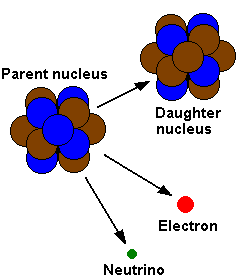β -particles are electrons moving at high speeds. These have greater (compared to α-particles) penetrating power but less ionizing power. Their emission velocity is almost the velocity of light. Unlike α-particles, they have a spectrum of energy, i.e., beta particles possess energy from a certain minimum to a certain maximum value. Usually, a β-particle is an electron, but can also be a positron. A positron is a positively charged particle which is the anti-matter equivalent of electron. In case, the process involves emission of an electron, it decreases the number of neutrons by one while the number of protons increases by one. Consider the following exampleBeta decay itself comes in two kinds; one is  positive β+ decay and another is β- decay.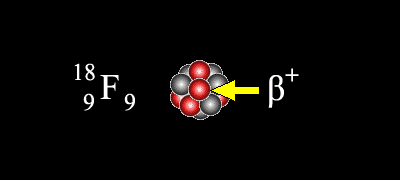β- emission occurs by the transformation of one of the nucleus’s neutrons into a proton, an electron and an antineutrino. The resultants or byproducts of fission from nuclear reactors experience β- decay as they have an excess of neutrons.

β+ decays is a similar process, but involves a proton changing into a neutron, a positron and a neutrino.

## Gamma RaysGamma rays are electromagnetic waves of wavelength of the order of ~ 10-12 m. This radioactive emission has the least ionization power. These are emitted due to transition of excited nucleus from higher energy state to lower energy state. Once an alpha or beta-decay occurs in a nucleus, it is often left in an excited state with excess energy. Just as an electron can move to a lower energy state by emitting a photon somewhere in the ultraviolet to infrared range, an atomic nucleus loses energy by emitting a gamma ray. The penetration power is maximum for gamma rays.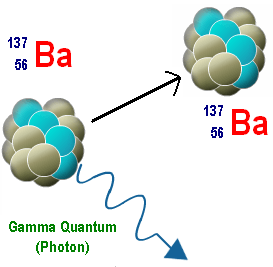The question that is generally asked in exams is which has the maximum penetration power- alpha or X-rays? We have already seen that alpha rays have the least penetration power. Gamma rays have the maximum penetration power, even more than X-rays. But since the question asks between alpha and X-rays, so the answer is X-rays.

Now, let us compare all the three rays on the basis of their penetration power.

Gamma radiation is the most penetrating of the three, and will travel through several centimeters of lead. Beta particles are absorbed by a few millimeters of aluminum but alpha particles will be stopped even by a few centimeters of air or a thin sheet of paper. Alpha rays cause the maximum destruction to the materials they touch.

The figure given below clearly describes the penetration power of the three:

###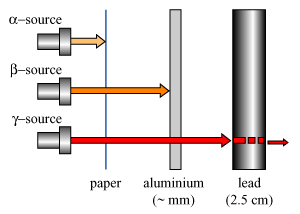Problem (JEE Main):-

N1 atoms of a radioactive element emit N2 beta particles per second. The decay constant of the element is (in s-1)

(a) N1/N           (b)  N2/N1

(c)N1 ln(2)         (c) Nln(2)

Solution:-

Activity of a radioactive substance R = λN

So,  λ = R/N

Here, R = N2

and N = N1

Thus, λ = N2/N1

From the above observation we conclude that, option (b) is correct.

Watch this Video for more reference

##• Life of all the radioactive elements is finite.

• Radioactive decay constant ‘’, of different elements are different.

• Elements having greater ‘’ have smaller half life.

• Each of the product of disintegration is a new element having physical and chemical properties different from those of the parent atom.

• Rate of disintegration of the radioactive substance, at any instant, is directly proportional to the number of atoms present at that instant. This is known as statistical law of radioactivity.

• A radioactive nucleus formed with an increase in (N-Z) and in consequence heavier than the known stable one tends to exhibit electron activity.

•  A radioactive nucleus formed with an decrease in (N-Z) and in consequence too light to be stable, tends to show a positron emission.Question 1(a) Curie                       (b) Rutherford

(c) Becquerel               (d) Roentgen

Question 2

The phenomenon of radioactivity is associated with:

(a) decay of the nucleus                                          (b) decay of the atom

(c) nuclear reaction caused by cosmic radiation     (d) fusion of the nucleus

Question 3An alpha particle has penetrating power compared to a beta particle:

(a) more                  (b) less

(c) same                 (d) none of the above

Question 4

‘Roentgen’ is the unit of :

Question 5

Which of the following is not a mode of radioactive decay?

(a) positron emission           (b) electron capture

(c) fusion                             (d) alpha decayQ.1 Q.2 Q.3 Q.4 Q.5 c a b d d

## Related Resources### Course Features

• Video Lectures
• Revision Notes
• Previous Year Papers
• Mind Map
• Study Planner
• NCERT Solutions
• Discussion Forum
• Test paper with Video Solution